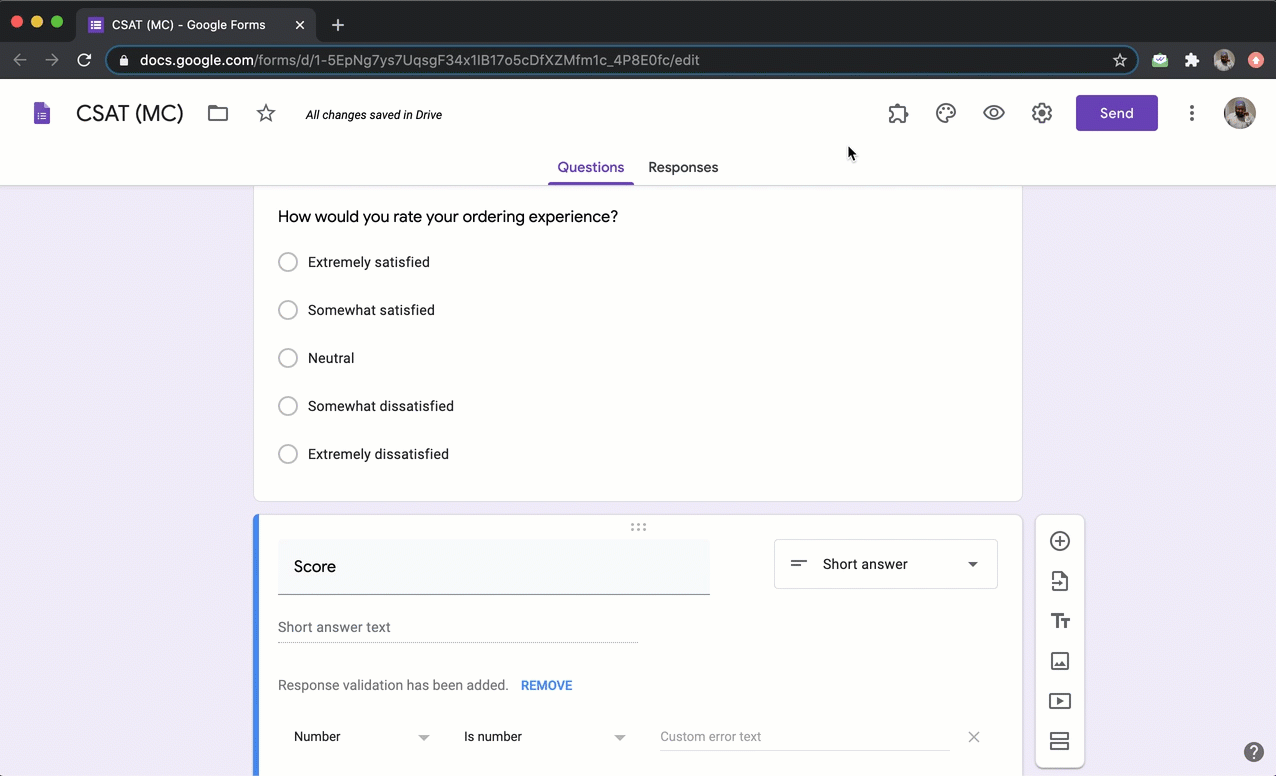### You can use the SCORE function to assign points for the answer choices in the multiple choice question and calculate the score.#### How to assign points using the SCORE function?

Let's create a simple form to collect feedback on ordering experience. We will use a multiple choice question with a 5 point likert scale for satisfaction as answer choices. Please follow the instructions below to assign points and calculate the score.#### SCORE function

The form is used to collect feedback from users about their ordering experience. A 5 point likert scale is used to measure customer satisfaction. You can assign points to the answer choices in multiple choice question using SCORE function.

Extremely satisfied = 5
Somewhat satisfied = 4
Neutral = 3
Somewhat dissatisfied = 2
Extremely dissatisfied = 1

SCORE(entry#id, 5, 4, 3, 2, 1)

Note: Type @ and select the required multiple choice question from the list to add its entry #id in the above formula.